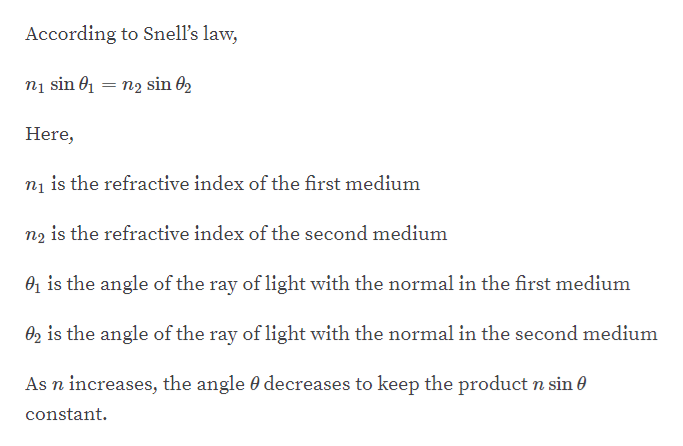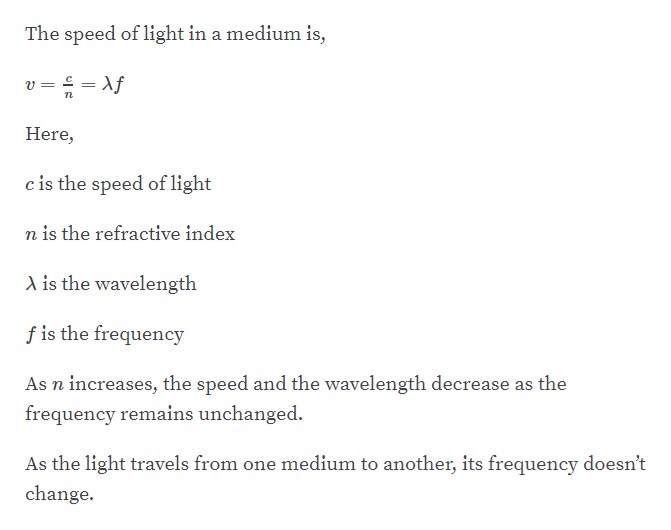# A ray of light passes from one material into a material witha higher index of refraction. Determine whether each ofthe following quantities increases, decreases, or remainsunchanged. Indicate your answers with I, D, or U, respectively.(a) The ray’s angle with the normal. (b) The light’swavelength. (c) The light’s frequency. (d) The light’s speed.(e) The photon energy.

Question
11 views

A ray of light passes from one material into a material with
a higher index of refraction. Determine whether each of
the following quantities increases, decreases, or remains
(a) The ray’s angle with the normal. (b) The light’s
wavelength. (c) The light’s frequency. (d) The light’s speed.
(e) The photon energy.

check_circle

Step 1help_outlineImage TranscriptioncloseAccording to Snell's law, nį sin 01 = n2 sin 02 %3D Here, ni is the refractive index of the first medium n2 is the refractive index of the second medium 01 is the angle of the ray of light with the normal in the first medium 02 is the angle of the ray of light with the normal in the second medium As n increases, the angle 0 decreases to keep the product n sin 0 constant. fullscreen
Step 2help_outlineImage TranscriptioncloseThe speed of light in a medium is, v = = Af Here, c is the speed of light n is the refractive index A is the wavelength f is the frequency As n increases, the speed and the wavelength decrease as the frequency remains unchanged. As the light travels from one medium to another, its frequency doesn't change. fullscreen

### Want to see the full answer?

See Solution

#### Want to see this answer and more?

Solutions are written by subject experts who are available 24/7. Questions are typically answered within 1 hour.*

See Solution
*Response times may vary by subject and question.
Tagged in

### Other